Posted by Samuel.Ogundiran on July 17, 2021

Unlike arrays Nodes, are pieces of data dispersed throughout the computer memory offering a new way to organize & access data yielding a performance boost. Linked List look and act like array on the surface, but tells a different story under the hood.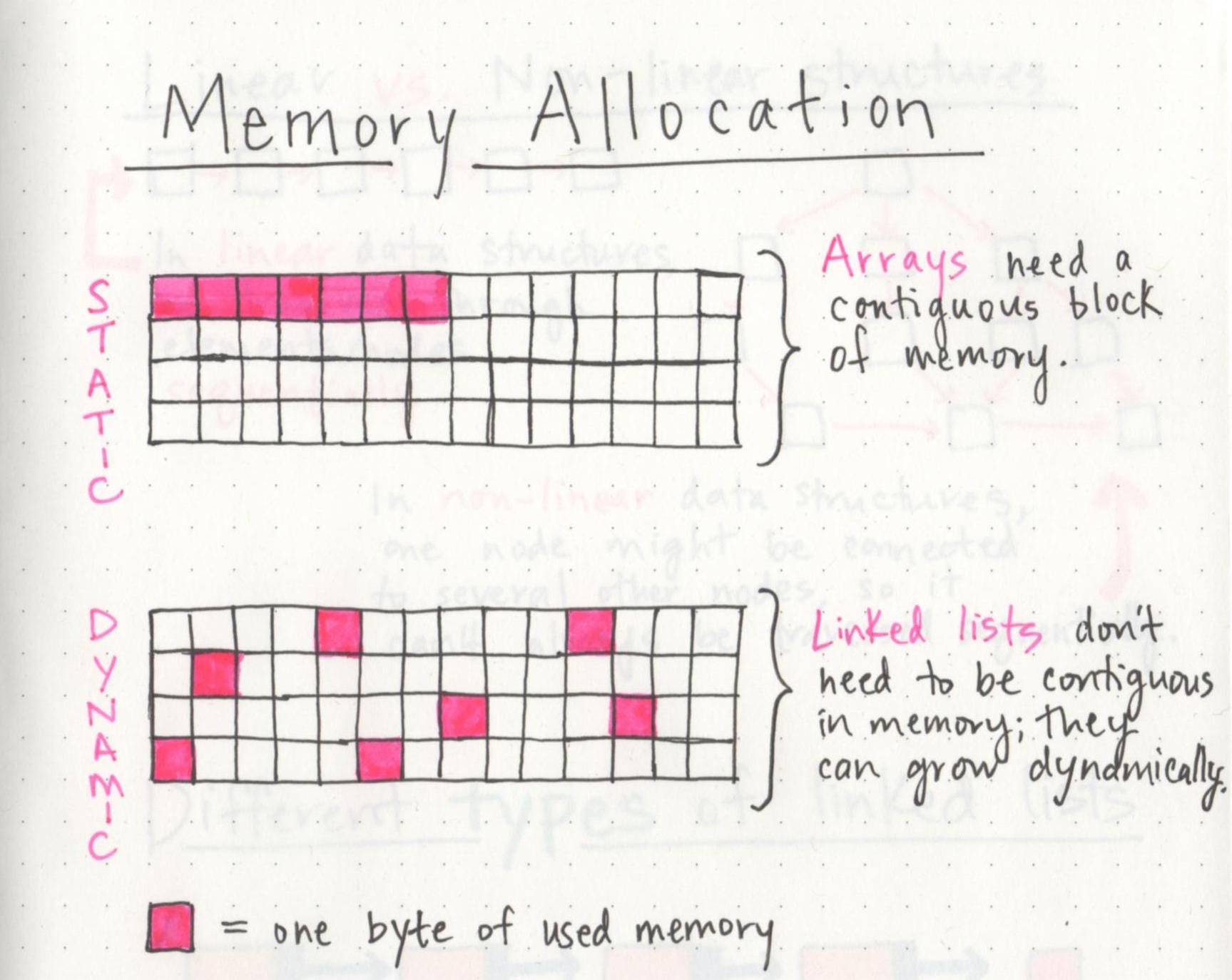The picture above illustrates that an Array Data Structure(ADS) need a contiguous block in memory, While Linked lists do not need a contiguous block of code in memory, thus has the possibility to grow dynamically. Simply put, data is scattered across different computer memory.

Pay attention to the above because it comes in handy later on

## Many flavors

In the Node world, Singly-Linked List and Doubly-linked list are the most popular of them all. Spending some time with algorithm and data structure , and you certainly have heard of this two brothers. Which begs the questions what makes them so special? .

## Singly- Linked list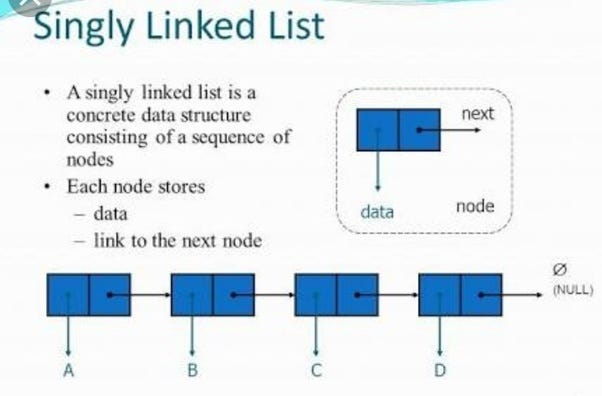As you can see, each box is connected with a line between them . The boxes are Nodes and the lines are called Links. The dispersed node in memory allows for nodes containing data information to be scattered across the memory because each node almost always contains the address of the node that comes after it. Making it possible for us to traverse the whole singly linked list because all that is required is the first node in the sequence formally known as the head.

Note: Languages such as Java come Prepackaged with LinkedList built in so you can do a lot with those.

**Code For Singly Linked List Implementation **

``````Ruby implementation.

class Node
attr_accessor :data, :next_node
def initialize(data)
@data  = data
end
end

Breakdown:
Node class has  two attributes
data-- contains the node's primary value(i.e the string "b")
next_node -- contains the link to the next node in the list.

So we have something like this.
node_1 = Node.new("once")
node_2 = Node.new("upon")
node_3 = Node.new("a")
node_4 = Node.new("time")

node_1.next_node = node_2
node_2.next_node = node_3
node_3.next_node = node_4

Note: we say "next_node" (next_node serve as the Node's Link), we dont need to specifiy memeory address, as the computer
keep track of that for us anyway.

Now we need to indicate where the node Start, So we create a LinkedList class which we will add to
our previous Node class.

attr_accessor :first_node

def initialize(first_node)
@first_node = first_node

# linkedList class is only keep track of the 1st Node. And we use the LinkedList class to reference the list by
writing this follow- up code

list = LinkedList.new(node_1) # this give access only to the first node.

end
end
``````

#### RISD(Read, Insert, Search, Delete) a Singly Linked List

Just like an array we can perform the read, insert, search and delete on the Singly linked list just in a different manner.

Reading is of O(N) time because the “Head” of this node is the only connection we have to the other nodes. Thus, the computer would go through each node to compute its values while also reading the address for the next node and then go to the location of the following node. This process repeats to the end of the SinglyLinked list.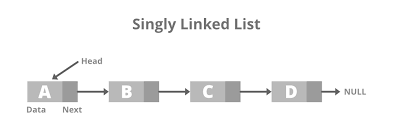** Code Implementation for Reading**

``````

# start with first Node of the list:
current_node = first_node
current_index = 0

while current _index < index do
#follow the links of eachh node until we get to the Index we're looking for:
current_node = current_node.next_node
current_index += 1

#if we get to the end of the list,=== we cannot found the value we are looking for
#so we return nil. the last node was never assigned a next_node of its own.

return nil unless current_node

end
return current_node.data
end

#how we call it,

``````

## Insert

LinkedList shines when inserting data compared to array because an Array(insertion is 0(n)) while singlyLinked list (insertion is 0(1)) making it super efficient. However, It get tricky. Inserting at the beginning or “head” of the list cost one step 0(1) as we do not need to shift any cells to make a run for the new data.

Inserting in the middle of an already existing singlyLink list data cost us 0(n) + 0(1) 0(n) to go through the to the specific node and locate the proper place for insertion, then 0(1) to conduct the insertion.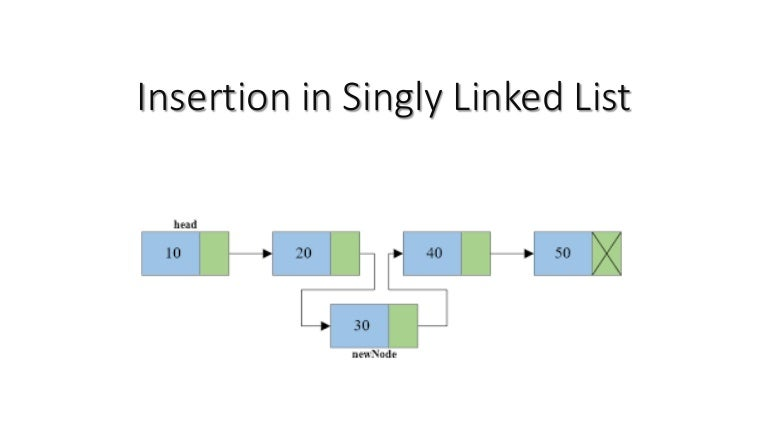** Code Implementation for Reading**

``````Ruby Implementation

def insert_at_index(index, value)

#we create the new Node with the provided value:
new_node = Node.new(value)

#if we are inserting at the beginning of the list:
if index == 0
new_node.next_node = first_node

#Establist that our new Node is now the list's first node:
self.first_node = new_node
return
end

#if we are inserting anywhere other than the beginning:
current_node = first_node
cuttent_index = 0

#first, we access the node immediately before where the new node will go:

while current_index < (index -1) do ## Draw out this part
current_node = current_node.next_node
current_index += 1
end

#Have the new Node link to the next node:
new_node.next_node = current_node.next_node

#modify the link of the previous node to the point to our new Node:
current_node.next_node = new_node
end

end

calling it

list.insert_at_index(2, "purple"). # insert the index where the new value should go
and data for the new value.
``````

Just like an array, the search speed for a singlyLinked list is 0(N). As we traverse through each node starting from the “head” node till we found the node we want too get to the end of our singly-linked list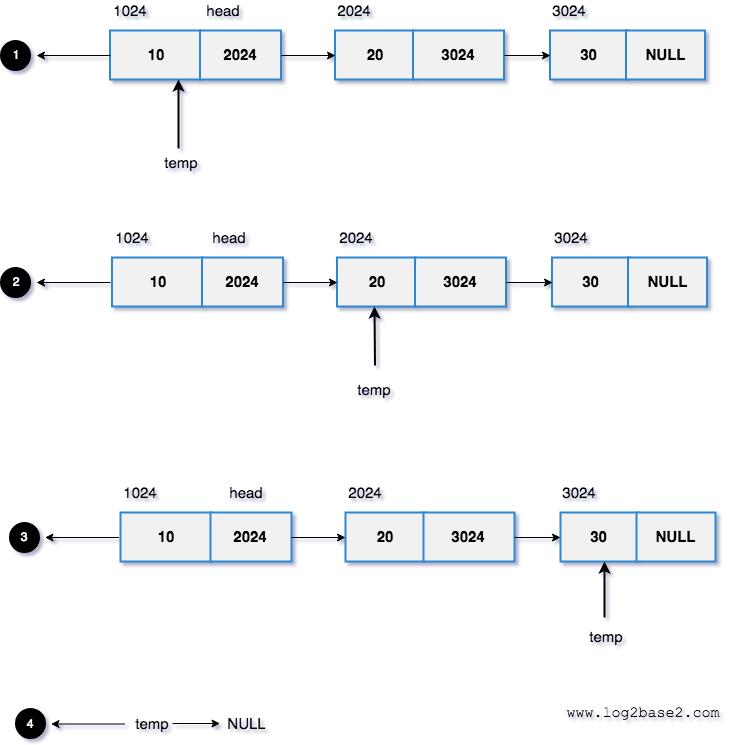Code for Searching

``````  def index_of(value)
# start with first Node of the list:
current_node = first_node
current_index = 0

begin
# if we find the data we are looking for,we return it.
if current_node.data == value
return current_index
end

#otherwise, we move to the next node:
current_node = current_node.next_node
current_index += 1
end while current_node

#if we get to the end of the list and cant find data or element, return nil
return nil
end

#how we call it,

list.index_of("time")

Note: it similar to the Reading function of linkedlist. Differences is that the loop doesn't
stop at a particular index, but run until either find the value or each the end of the list.

``````

## Delete

Just like the insert, It get tricky.

Deleting at the beginning or “head” of the list costs one step 0(1) as we do not need to shift any cells to make a room for the new data.

Deleting in the middle or end of an already existing singlyLink list data cost us 0(n) + 0(1) 0(n) to go through the exciting and locate the proper place for deletion, then 0(1) to conduct the deletion. So we just say 0(n) for deletion

Fun Fact on LinkedList deletion:

Its interesting to note that whenever we delete a node from a linked list, the node still exists in memory somewhere. We just remove the node from our list ensuring no other node links to it. Which gives the effect of deleting the node from our list, even if the node still exists in memory. Some program automatically detect that they’re not being used and will “garage collect” them and Free up memory**Code for Deletion **

``````Code Implementation: Linked list deletion

ruby implementation

def delete_at_index(index)

#if we are deleting the 1st node
if index == 0
#simply set the first node to be what is currently the seconde node:
self.first_node = first_node.next_node
end

current_node = first_node
current_index = 0

#first, we find the node before the one we wwant to delete and call it current node
while current_index < (index - 1) do
current_node = current_node.next_node
current_index += 1
end

#We find node that comes after the node that we are deleting.
node_after_deleted_node = current_node.next_node.next_node

#we change the link of current_node to point to the Node_after_deleted_node,
#leaving the node we want to delete out of the list:

current_node.next_node = node_after_deleted_node

end

``````

Link below expand on this topic with basic level depth.